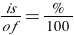# Percent Word Problems

In this lesson, we will be working with percents. If you have worked with fractions and know what a percent is, you already have a good base for this lesson. Think about percents and what they mean as a fraction. What does 25% mean? The word “percent” means “per 100.” If you remember it means 20 per 100 or 25 out of 100 or 25/100. A percent is a ratio.Since percents can be written as ratios, we can say that percent problems can also be worked using proportions.

All percent problems fit the proportion:One could say that 40% of 60 is 24 . Placing these numbers into the proportion gives usRemember, percent means out of 100, so the denominator of the percent ratio will always be 100. If one of the parts of the proportion is missing, we use a letter to represent the unknown. Then we can cross multiply to find our unknown portion.

Let’s take a look at a problem where we need to find some information. We use percents in our everyday life such as a sale on clothes at department stores, the percent of games won, and surveys.

### Example 1:

At the department store, we find a pair of shoes on sale. We want to make sure we have enough to buy them. According to the sign the price of the shoes is 80% of the full price of \$56. What is the sale price?

Step 1:

Set up of the proportion:Step 2:

Use the cross product property to solve for x!!  In other words, cross multiply.

100x = 4480

x = 44.8

“A percent of a number is another number.”   This phrase can be used to solve percent word problems.   The word “of” in math means to multiply.   The word “is” in math means equal. So you should multiply the percent (written as a decimal) times the given number.   The answer is the product.

### Example 2

Sales tax is 5% in Maryland.   Jim bought a pair of basketball shoes advertised for \$62.   How much sales tax did he pay?

Step 1:

Convert your percent to a decimal.

5% = 0.05 (move the decimal two places to the left)

Step 2:

Multiply the decimal by the price.

0.05 times \$62 equals the Maryland sales tax

0.05 (62) = ?

The sales tax was \$3.10 in Maryland.

## Solve

1. You surveyed 10 people. Six of them prefer lemonade to water. Write the number of people who prefer lemonade as a decimal.

2. Write 145% as a decimal.

3. Write .25 as both a fraction and a percent.

4. Write 220% as a fraction. Leave your answer in lowest term and as a mixed number. (Hint: Write as a decimal first.)

5. At Leslie’s Pizza Place, 85% of the pizzas sold were pepperoni. At Adriana’s Pizza, 41 out of 48 pizzas sold were pepperoni. Which pizza parlor sold a greater percentage of pepperoni pizza?

6. Last week, 1/5 of the mice in Adriana’s lab found the cheese at the end of a maze. In Leslie’s lab, 23% of the mice found the cheese at the end of a maze. Which lab had a greater percentage of mice who found the cheese?